# GED Math : Radius and Diameter

## Example Questions

### Example Question #11 : Geometry And Graphs

Determine the diameter if the radius is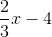.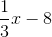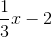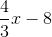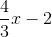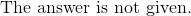Explanation:

The diameter is double the radius.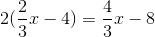The answer is:### Example Question #12 : Geometry And Graphs

Determine the diameter of a circle if the radius is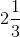.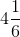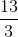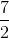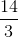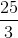Explanation:

The diameter is twice the radius.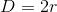Convert the mixed fraction to an improper fraction.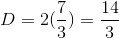The answer is:### Example Question #13 : Geometry And Graphs

Determine the diameter if the radius is.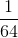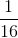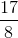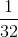Explanation:

The diameter is twice the radius.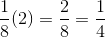The answer is:### Example Question #14 : Geometry And Graphs

What is the radius if the diameter of a circle is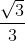?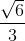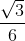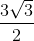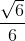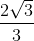Explanation:

The radius is half the diameter of a circle.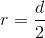This is also similar to multiply diameter by one-half.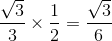The answer is:### Example Question #15 : Geometry And Graphs

What is the radius with a diameter of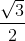?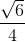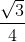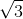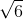Explanation:

The radius is half the diameter.

Multiply the diameter by one-half.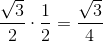The answer is:### Example Question #16 : Geometry And Graphs

Find the radius of a circle with an area of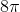.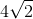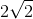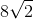Explanation:

Write the formula for the area of a circle.Substitute the area.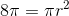Divide by pi on both sides.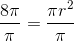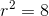Square root both sides.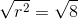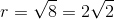The answer is:### Example Question #17 : Geometry And Graphs

Find the value of the diameter if the radius is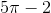.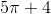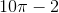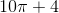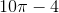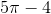Explanation:

The diameter is double the radius.

Multiply the quantity by two.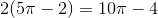The answer is:### Example Question #18 : Geometry And Graphs

Determine the radius if the diameter is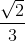.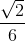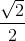Explanation:

The radius is half the diameter.

Multiply the diameter by half.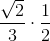The answer is:### Example Question #19 : Geometry And Graphs

Determine the radius of the circle if the diameter is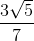.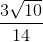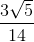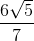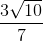Explanation:

The radius is half the diameter.  To find the radius, multiply the diameter by half.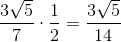The answer is:### Example Question #20 : Geometry And Graphs

Determine the radius of the circle if the diameter of a circle is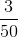.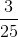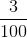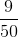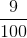Explanation:

The radius is half the diameter.

Multiply the diameter by one-half.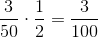The answer is: